xGibbs free energyEncyclopedia
In thermodynamics
Thermodynamics
Thermodynamics is a physical science that studies the effects on material bodies, and on radiation in regions of space, of transfer of heat and of work done on or by the bodies or radiation...

, the Gibbs free energy (IUPAC recommended name: Gibbs energy or Gibbs function; also known as free enthalpy to distinguish it from Helmholtz free energy
Helmholtz free energy
In thermodynamics, the Helmholtz free energy is a thermodynamic potential that measures the “useful” work obtainable from a closed thermodynamic system at a constant temperature and volume...

) is a thermodynamic potential that measures the "useful" or process-initiating work
Work (thermodynamics)
In thermodynamics, work performed by a system is the energy transferred to another system that is measured by the external generalized mechanical constraints on the system. As such, thermodynamic work is a generalization of the concept of mechanical work in mechanics. Thermodynamic work encompasses...

obtainable from a thermodynamic system
Thermodynamic system
A thermodynamic system is a precisely defined macroscopic region of the universe, often called a physical system, that is studied using the principles of thermodynamics....

at a constant temperature and pressure (isothermal, isobaric
Isobaric process
An isobaric process is a thermodynamic process in which the pressure stays constant. The term derives from the Greek isos, , and barus,...

). Just as in mechanics
Mechanics
Mechanics is the branch of physics concerned with the behavior of physical bodies when subjected to forces or displacements, and the subsequent effects of the bodies on their environment....

, where potential energy
Potential energy
In physics, potential energy is the energy stored in a body or in a system due to its position in a force field or due to its configuration. The SI unit of measure for energy and work is the Joule...

is defined as capacity to do work, similarly different potentials have different meanings. Gibbs energy is the capacity of a system to do non-mechanical work and ΔG measures the non-mechanical work done on it. The Gibbs free energy is the maximum amount of non-expansion work that can be extracted from a closed system
Closed system
-In physics:In thermodynamics, a closed system can exchange energy , but not matter, with its surroundings.In contrast, an isolated system cannot exchange any of heat, work, or matter with the surroundings, while an open system can exchange all of heat, work and matter.For a simple system, with...

; this maximum can be attained only in a completely reversible process
Reversible process (thermodynamics)
In thermodynamics, a reversible process, or reversible cycle if the process is cyclic, is a process that can be "reversed" by means of infinitesimal changes in some property of the system without loss or dissipation of energy. Due to these infinitesimal changes, the system is in thermodynamic...

. When a system changes from a well-defined initial state to a well-defined final state, the Gibbs free energy ΔG equals the work exchanged by the system with its surroundings, minus the work of the pressure
Pressure
Pressure is the force per unit area applied in a direction perpendicular to the surface of an object. Gauge pressure is the pressure relative to the local atmospheric or ambient pressure.- Definition :...

forces, during a reversible transformation of the system from the same initial state to the same final state.

Gibbs energy (also referred to as ∆G) is also the chemical potential that is minimized when a system reaches equilibrium at constant pressure and temperature. Its derivative with respect to the reaction coordinate of the system vanishes at the equilibrium point. As such, it is a convenient criterion of spontaneity for processes with constant pressure and temperature.

The Gibbs free energy, originally called available energy, was developed in the 1870s by the American mathematician Josiah Willard Gibbs
Josiah Willard Gibbs
Josiah Willard Gibbs was an American theoretical physicist, chemist, and mathematician. He devised much of the theoretical foundation for chemical thermodynamics as well as physical chemistry. As a mathematician, he invented vector analysis . Yale University awarded Gibbs the first American Ph.D...

. In 1873, Gibbs described this “available energy” as
The initial state of the body, according to Gibbs, is supposed to be such that "the body can be made to pass from it to states of dissipated energy by reversible processes
Reversible process (thermodynamics)
In thermodynamics, a reversible process, or reversible cycle if the process is cyclic, is a process that can be "reversed" by means of infinitesimal changes in some property of the system without loss or dissipation of energy. Due to these infinitesimal changes, the system is in thermodynamic...

." In his 1876 magnum opus
Magnum opus
Magnum opus , from the Latin meaning "great work", refers to the largest, and perhaps the best, greatest, most popular, or most renowned achievement of a writer, artist, or composer.-Related terms:Sometimes the term magnum opus is used to refer to simply "a great work" rather than "the...

On the Equilibrium of Heterogeneous Substances
On the Equilibrium of Heterogeneous Substances
In the history of thermodynamics, On the Equilibrium of Heterogeneous Substances is a 300-page paper written by American mathematical-engineer Willard Gibbs...

, a graphical analysis of multi-phase chemical systems, he engaged his thoughts on chemical free energy in full.

## Overview

In a simple manner, with respect to STP reacting systems, a general rule of thumb
Rule of thumb
A rule of thumb is a principle with broad application that is not intended to be strictly accurate or reliable for every situation. It is an easily learned and easily applied procedure for approximately calculating or recalling some value, or for making some determination...

is:
Hence, out of this general natural tendency, a quantitative measure as to how near or far a potential reaction is from this minimum is when the calculated energetics of the process indicate that the change in Gibbs free energy ΔG is negative. In essence, this means that such a reaction will be favoured and will release energy. The energy released equals the maximum amount of work that can be performed as a result of the chemical reaction. In contrast, if conditions indicated a positive ΔG, then energy—in the form of work—would have to be added to the reacting system to make the reaction go.

The equation can also be seen from the perspective of both the system and its surroundings (the universe). For the purposes of calculation, we assume the reaction is the only reaction going on in the universe. Thus the entropy released or absorbed by the system is actually the entropy that the environment must absorb or release respectively. Thus the reaction will only be allowed if the total entropy change of the universe is equal to zero (an equilibrium process) or positive. The input of heat into an "endothermic" chemical reaction (e.g. the elimination of cyclohexanol
Cyclohexanol
Cyclohexanol is the organic compound with the formula 5CHOH. The molecule is related to cyclohexane ring by replacement of one hydrogen atom by a hydroxyl group. This compound exists as a deliquescent colorless solid, which, when very pure, melts near room temperature...

to cyclohexene
Cyclohexene
Cyclohexene is a hydrocarbon with the formula C6H10. This cycloalkene is a colorless liquid with a sharp smell. It is an intermediate in various industrial processes...

) can be seen as coupling an inherently unfavourable reaction (elimination) to a favourable one (burning of coal or the energy source of a heat source) such that the total entropy change of the universe is more than or equal to zero, making the Gibbs free energy of the coupled reaction negative.

In traditional use, the term “free” was attached to Gibbs free energy for systems at constant pressure and temperature to mean "available in the form of useful work." For Gibbs free energy, we add the qualification that it is the energy free for non-volume work. (A similar meaning applies used in conjunction with Helmholtz free energy, for systems at constant volume and temperature). However, an increasing number of books and journal articles do not include the attachment “free”, referring to G as simply "Gibbs energy". This is the result of a 1988 IUPAC meeting to set unified terminologies for the international scientific community, in which the adjective ‘free’ was supposedly banished. This standard, however, has not yet been universally adopted.

## History

The quantity called "free energy" is a more advanced and accurate replacement for the outdated term affinity, which was used by chemists in previous years to describe the force that caused chemical reaction
Chemical reaction
A chemical reaction is a process that leads to the transformation of one set of chemical substances to another. Chemical reactions can be either spontaneous, requiring no input of energy, or non-spontaneous, typically following the input of some type of energy, such as heat, light or electricity...

s.

In 1873, Willard Gibbs published A Method of Geometrical Representation of the Thermodynamic Properties of Substances by Means of Surfaces, in which he introduced the preliminary outline of the principles of his new equation able to predict or estimate the tendencies of various natural processes to ensue when bodies or systems are brought into contact. By studying the interactions of homogeneous substances in contact, i.e., bodies, being in composition part solid, part liquid, and part vapor, and by using a three-dimensional volume-entropy
Entropy
Entropy is a thermodynamic property that can be used to determine the energy available for useful work in a thermodynamic process, such as in energy conversion devices, engines, or machines. Such devices can only be driven by convertible energy, and have a theoretical maximum efficiency when...

-internal energy
Internal energy
In thermodynamics, the internal energy is the total energy contained by a thermodynamic system. It is the energy needed to create the system, but excludes the energy to displace the system's surroundings, any energy associated with a move as a whole, or due to external force fields. Internal...

graph, Gibbs was able to determine three states of equilibrium, i.e., "necessarily stable", "neutral", and "unstable", and whether or not changes would ensue.

Hence, in 1882, the German scientist Hermann von Helmholtz
Hermann von Helmholtz
Hermann Ludwig Ferdinand von Helmholtz was a German physician and physicist who made significant contributions to several widely varied areas of modern science...

stated that affinity is the largest quantity of work which can be gained when the reaction is carried out in a reversible manner, e.g., electrical work in a reversible cell. The maximum work is thus regarded as the diminution of the free, or available, energy of the system (Gibbs free energy G at T = constant, P = constant or Helmholtz free energy F at T = constant, V = constant), whilst the heat given out is usually a measure of the diminution of the total energy of the system (Internal energy
Internal energy
In thermodynamics, the internal energy is the total energy contained by a thermodynamic system. It is the energy needed to create the system, but excludes the energy to displace the system's surroundings, any energy associated with a move as a whole, or due to external force fields. Internal...

). Thus, G or F is the amount of energy “free” for work under the given conditions.

Up until this point, the general view had been such that: “all chemical reactions drive the system to a state of equilibrium in which the affinities of the reactions vanish”. Over the next 60 years, the term affinity came to be replaced with the term free energy. According to chemistry historian Henry Leicester, the influential 1923 textbook Thermodynamics and the Free Energy of Chemical Reactions by Gilbert N. Lewis
Gilbert N. Lewis
Gilbert Newton Lewis was an American physical chemist known for the discovery of the covalent bond , his purification of heavy water, his reformulation of chemical thermodynamics in a mathematically rigorous manner accessible to ordinary chemists, his theory of Lewis acids and...

and Merle Randall
Merle Randall
Merle Randall was an American physical chemist famous for his work, over the period of 25 years, in measuring free energy calculations of compounds with Gilbert N. Lewis...

led to the replacement of the term “affinity” by the term “free energy” in much of the English-speaking world.

## Graphical interpretation

Gibbs free energy was originally defined graphically. In 1873, American engineer Willard Gibbs published his first thermodynamics paper, “Graphical Methods in the Thermodynamics of Fluids”, in which Gibbs used the two coordinates of the entropy and volume to represent the state of the body. In his second follow-up paper, “A Method of Geometrical Representation of the Thermodynamic Properties of Substances by Means of Surfaces”, published later that year, Gibbs added in the third coordinate of the energy of the body, defined on three figures. In 1874, Scottish physicist James Clerk Maxwell
James Clerk Maxwell
James Clerk Maxwell of Glenlair was a Scottish physicist and mathematician. His most prominent achievement was formulating classical electromagnetic theory. This united all previously unrelated observations, experiments and equations of electricity, magnetism and optics into a consistent theory...

used Gibbs' figures to make a 3D energy-entropy-volume thermodynamic surface
Maxwell's thermodynamic surface
Maxwell’s thermodynamic surface is an 1874 sculpture made by Scottish physicist James Clerk Maxwell . This model provides a three-dimensional plot of the various states of a fictitious substance with water-like properties. This plot has coordinates volume , entropy , and energy...

of a fictitious water-like substance. Thus, in order to understand the very difficult concept of Gibbs free energy one must be able to understand its interpretation as Gibbs defined originally by section AB on his figure 3 and as Maxwell sculpted that section on his 3D surface figure
Maxwell's thermodynamic surface
Maxwell’s thermodynamic surface is an 1874 sculpture made by Scottish physicist James Clerk Maxwell . This model provides a three-dimensional plot of the various states of a fictitious substance with water-like properties. This plot has coordinates volume , entropy , and energy...

.

## DefinitionsThe Gibbs free energy is defined as:
G(p,T) = U + pVTS

which is the same as:
G(p,T) = HTS

where:
• U is the internal energy
Internal energy
In thermodynamics, the internal energy is the total energy contained by a thermodynamic system. It is the energy needed to create the system, but excludes the energy to displace the system's surroundings, any energy associated with a move as a whole, or due to external force fields. Internal...

(SI
Si
Si, si, or SI may refer to :- Measurement, mathematics and science :* International System of Units , the modern international standard version of the metric system...

unit: joule
Joule
The joule ; symbol J) is a derived unit of energy or work in the International System of Units. It is equal to the energy expended in applying a force of one newton through a distance of one metre , or in passing an electric current of one ampere through a resistance of one ohm for one second...

)
• p is pressure
Pressure
Pressure is the force per unit area applied in a direction perpendicular to the surface of an object. Gauge pressure is the pressure relative to the local atmospheric or ambient pressure.- Definition :...

(SI unit: pascal
Pascal (unit)
The pascal is the SI derived unit of pressure, internal pressure, stress, Young's modulus and tensile strength, named after the French mathematician, physicist, inventor, writer, and philosopher Blaise Pascal. It is a measure of force per unit area, defined as one newton per square metre...

)
• V is volume (SI unit: m3)
• T is the temperature
Temperature
Temperature is a physical property of matter that quantitatively expresses the common notions of hot and cold. Objects of low temperature are cold, while various degrees of higher temperatures are referred to as warm or hot...

(SI unit: kelvin
Kelvin
The kelvin is a unit of measurement for temperature. It is one of the seven base units in the International System of Units and is assigned the unit symbol K. The Kelvin scale is an absolute, thermodynamic temperature scale using as its null point absolute zero, the temperature at which all...

)
• S is the entropy (SI unit: joule per kelvin)
• H is the enthalpy
Enthalpy
Enthalpy is a measure of the total energy of a thermodynamic system. It includes the internal energy, which is the energy required to create a system, and the amount of energy required to make room for it by displacing its environment and establishing its volume and pressure.Enthalpy is a...

(SI unit: joule)

The expression for the infinitesimal reversible change in the Gibbs free energy as a function of its 'natural variables' p and T, for an open system, subjected to the operation of external forces (for instance electrical or magnetical) Xi, which cause the external parameters of the system ai to change by an amount dai, can be derived as follows from the First Law for reversible processes: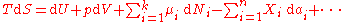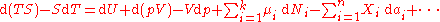where:
• μi is the chemical potential
Chemical potential
Chemical potential, symbolized by μ, is a measure first described by the American engineer, chemist and mathematical physicist Josiah Willard Gibbs. It is the potential that a substance has to produce in order to alter a system...

of the ith chemical component
Chemical species
Chemical species are atoms, molecules, molecular fragments, ions, etc., being subjected to a chemical process or to a measurement. Generally, a chemical species can be defined as an ensemble of chemically identical molecular entities that can explore the same set of molecular energy levels on a...

. (SI unit: joules per particle or joules per mole)
• Ni is the number of particles
Particle number
The particle number of a thermodynamic system, conventionally indicated with the letter N, is the number of constituent particles in that system. The particle number is a fundamental parameter in thermodynamics which is conjugate to the chemical potential. Unlike most physical quantities, particle...

(or number of moles) composing the ith chemical component.

This is one form of Gibbs fundamental equation. In the infinitesimal expression, the term involving the chemical potential accounts for changes in Gibbs free energy resulting from an influx or outflux of particles. In other words, it holds for an open system
Open system
Open system may refer to:*Open system , one of a class of computers and associated software that provides some combination of interoperability, portability and open software standards, particularly Unix and Unix-like systems...

. For a closed system
Closed system
-In physics:In thermodynamics, a closed system can exchange energy , but not matter, with its surroundings.In contrast, an isolated system cannot exchange any of heat, work, or matter with the surroundings, while an open system can exchange all of heat, work and matter.For a simple system, with...

, this term may be dropped.

Any number of extra terms may be added, depending on the particular system being considered. Aside from mechanical work
Mechanical work
In physics, work is a scalar quantity that can be described as the product of a force times the distance through which it acts, and it is called the work of the force. Only the component of a force in the direction of the movement of its point of application does work...

, a system may, in addition, perform numerous other types of work. For example, in the infinitesimal expression, the contractile work energy associated with a thermodynamic system that is a contractile fiber that shortens by an amount −dl under a force f would result in a term fdl being added. If a quantity of charge −de is acquired by a system at an electrical potential Ψ, the electrical work associated with this is −Ψde, which would be included in the infinitesimal expression. Other work terms are added on per system requirements.

Each quantity in the equations above can be divided by the amount of substance, measured in moles
Mole (unit)
The mole is a unit of measurement used in chemistry to express amounts of a chemical substance, defined as an amount of a substance that contains as many elementary entities as there are atoms in 12 grams of pure carbon-12 , the isotope of carbon with atomic weight 12. This corresponds to a value...

, to form molar Gibbs free energy. The Gibbs free energy is one of the most important thermodynamic functions for the characterization of a system. It is a factor in determining outcomes such as the voltage
Voltage
Voltage, otherwise known as electrical potential difference or electric tension is the difference in electric potential between two points — or the difference in electric potential energy per unit charge between two points...

of an electrochemical cell
Electrochemical cell
An electrochemical cell is a device capable of either deriving electrical energy from chemical reactions, or facilitating chemical reactions through the introduction of electrical energy. A common example of an electrochemical cell is a standard 1.5-volt "battery"...

, and the equilibrium constant for a reversible reaction
Reversible reaction
A reversible reaction is a chemical reaction that results in an equilibrium mixture of reactants and products. For a reaction involving two reactants and two products this can be expressed symbolically as...

. In isothermal, isobaric systems, Gibbs free energy can be thought of as a "dynamic" quantity, in that it is a representative measure of the competing effects of the enthalpic and entropic driving forces involved in a thermodynamic process.

The temperature dependence of the Gibbs energy for an ideal gas
Ideal gas
An ideal gas is a theoretical gas composed of a set of randomly-moving, non-interacting point particles. The ideal gas concept is useful because it obeys the ideal gas law, a simplified equation of state, and is amenable to analysis under statistical mechanics.At normal conditions such as...

is given by the Gibbs-Helmholtz equation
Gibbs-Helmholtz equation
The Gibbs–Helmholtz equation is a thermodynamic equation useful for calculating changes in the Gibbs energy of a system as a function of temperature...

and its pressure dependence is given by: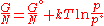if the volume is known rather than pressure then it becomes: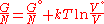or more conveniently as its chemical potential
Chemical potential
Chemical potential, symbolized by μ, is a measure first described by the American engineer, chemist and mathematical physicist Josiah Willard Gibbs. It is the potential that a substance has to produce in order to alter a system...

: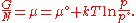In non-ideal systems, fugacity
Fugacity
In chemical thermodynamics, the fugacity of a real gas is an effective pressure which replaces the true mechanical pressure in accurate chemical equilibrium calculations. It is equal to the pressure of an ideal gas which has the same chemical potential as the real gas. For example, nitrogen gas ...

comes into play.

## Derivation

The Gibbs free energy total differential natural variables may be derived via Legendre transforms of the internal energy
Internal energy
In thermodynamics, the internal energy is the total energy contained by a thermodynamic system. It is the energy needed to create the system, but excludes the energy to displace the system's surroundings, any energy associated with a move as a whole, or due to external force fields. Internal...

..

Because S, V, and Ni are extensive variables
Intensive and extensive properties
In the physical sciences, an intensive property , is a physical property of a system that does not depend on the system size or the amount of material in the system: it is scale invariant.By contrast, an extensive property In the physical sciences, an intensive property (also called a bulk...

, Euler's homogeneous function theorem
Homogeneous function
In mathematics, a homogeneous function is a function with multiplicative scaling behaviour: if the argument is multiplied by a factor, then the result is multiplied by some power of this factor. More precisely, if is a function between two vector spaces over a field F, and k is an integer, then...

allows easy integration of dU: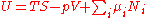.

The definition of G from above is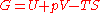.

Taking the total differential, we have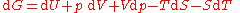.

Replacing dU with the result from the first law gives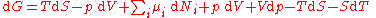.

The natural variables of G are then p, T, and {Ni}. Because some of the natural variables are intensive, dG may not be integrated using Euler integrals as is the case with internal energy. However, simply substituting the result for U into the definition of G gives a standard expression for G: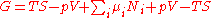.

## Free energy of reactions

To derive the Gibbs free energy equation for an isolated system
Isolated system
In the natural sciences an isolated system, as contrasted with an open system, is a physical system without any external exchange. If it has any surroundings, it does not interact with them. It obeys in particular the first of the conservation laws: its total energy - mass stays constant...

, let Stot be the total entropy of the isolated system
Isolated system
In the natural sciences an isolated system, as contrasted with an open system, is a physical system without any external exchange. If it has any surroundings, it does not interact with them. It obeys in particular the first of the conservation laws: its total energy - mass stays constant...

, that is, a system that cannot exchange heat or mass with its surroundings. According to the second law of thermodynamics
Second law of thermodynamics
The second law of thermodynamics is an expression of the tendency that over time, differences in temperature, pressure, and chemical potential equilibrate in an isolated physical system. From the state of thermodynamic equilibrium, the law deduced the principle of the increase of entropy and...

: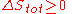and if ΔStot = 0 then the process is reversible. The heat transfer Q vanishes for an adiabatic system. Any adiabatic process
In thermodynamics, an adiabatic process or an isocaloric process is a thermodynamic process in which the net heat transfer to or from the working fluid is zero. Such a process can occur if the container of the system has thermally-insulated walls or the process happens in an extremely short time,...

that is also reversible is called an isentropic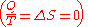process.

Now consider systems, having internal entropy Sint. Such a system is thermally connected to its surroundings, which have entropy Sext. The entropy form of the second law applies only to the closed system formed by both the system and its surroundings. Therefore a process is possible if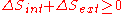.

If Q is heat transferred to the system from the surroundings, so −Q is heat lost by the surroundings
so that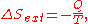corresponds to entropy change of the surroundings.

We now have: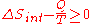Multiply both sides by T: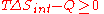Q is heat transferred to the system; if the process is now assumed to be isobaric
Isobaric process
An isobaric process is a thermodynamic process in which the pressure stays constant. The term derives from the Greek isos, , and barus,...

, then Qp = ΔH:ΔH is the enthalpy change of reaction (for a chemical reaction at constant pressure). Then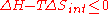for a possible process. Let the change ΔG in Gibbs free energy be defined as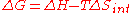(eq.1)
Notice that it is not defined in terms of any external state functions, such as ΔSext or ΔStot. Then the second law becomes, which also tells us about the spontaneity of the reaction: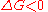favoured reaction (Spontaneous)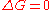Neither the forward nor the reverse reaction prevails (Equilibrium
Chemical equilibrium
In a chemical reaction, chemical equilibrium is the state in which the concentrations of the reactants and products have not yet changed with time. It occurs only in reversible reactions, and not in irreversible reactions. Usually, this state results when the forward reaction proceeds at the same...

)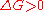disfavoured reaction (Nonspontaneous)

Gibbs free energy G itself is defined as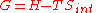(eq.2)
but notice that to obtain equation (2) from equation (1) we must assume that T is constant. Thus, Gibbs free energy is most useful for thermochemical processes at constant temperature and pressure: both isothermal and isobaric. Such processes don't move on a P-V diagram, such as phase change of a pure substance, which takes place at the saturation pressure and temperature. Chemical reactions, however, do undergo changes in chemical potential
Chemical potential
Chemical potential, symbolized by μ, is a measure first described by the American engineer, chemist and mathematical physicist Josiah Willard Gibbs. It is the potential that a substance has to produce in order to alter a system...

, which is a state function. Thus, thermodynamic processes are not confined to the two dimensional P-V diagram. There is a third dimension for n, the quantity of gas. For the study of explosive chemicals, the processes are not necessarily isothermal and isobaric. For these studies, Helmholtz free energy is used.

If an isolated system (Q = 0) is at constant pressure (Q = ΔH), then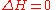Therefore the Gibbs free energy of an isolated system is: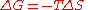and if ΔG ≤ 0 then this implies that ΔS ≥ 0, back to where we started the derivation of ΔG

## Useful identities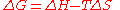for constant temperature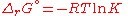(see Chemical equilibrium
Chemical equilibrium
In a chemical reaction, chemical equilibrium is the state in which the concentrations of the reactants and products have not yet changed with time. It occurs only in reversible reactions, and not in irreversible reactions. Usually, this state results when the forward reaction proceeds at the same...

).and rearranging gives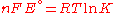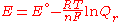which relates the electrical potential of a reaction to the equilibrium coefficient for that reaction (Nernst equation
Nernst equation
In electrochemistry, the Nernst equation is an equation that can be used to determine the equilibrium reduction potential of a half-cell in an electrochemical cell. It can also be used to determine the total voltage for a full electrochemical cell...

).

where

ΔG = change in Gibbs free energy, ΔH = change in enthalpy
Enthalpy
Enthalpy is a measure of the total energy of a thermodynamic system. It includes the internal energy, which is the energy required to create a system, and the amount of energy required to make room for it by displacing its environment and establishing its volume and pressure.Enthalpy is a...

, T = absolute temperature
Temperature
Temperature is a physical property of matter that quantitatively expresses the common notions of hot and cold. Objects of low temperature are cold, while various degrees of higher temperatures are referred to as warm or hot...

, ΔS = change in entropy
Entropy
Entropy is a thermodynamic property that can be used to determine the energy available for useful work in a thermodynamic process, such as in energy conversion devices, engines, or machines. Such devices can only be driven by convertible energy, and have a theoretical maximum efficiency when...

, R = gas constant
Gas constant
The gas constant is a physical constant which is featured in many fundamental equations in the physical sciences, such as the ideal gas law and the Nernst equation. It is equivalent to the Boltzmann constant, but expressed in units of energy The gas constant (also known as the molar, universal,...

, ln = natural logarithm
Natural logarithm
The natural logarithm is the logarithm to the base e, where e is an irrational and transcendental constant approximately equal to 2.718281828...

, ΔrG = change of reaction in Gibbs free energy, Δr = standard change of reaction in Gibbs free energy, K = equilibrium constant, Qr = reaction quotient
Reaction quotient
In chemistry, a reaction quotient: Qr is a function of the activities or concentrations of the chemical species involved in a chemical reaction. In the special case that the reaction is at equilibrium the reaction quotient is equal to the equilibrium constant....

, n = number of electrons per mole
Mole (unit)
The mole is a unit of measurement used in chemistry to express amounts of a chemical substance, defined as an amount of a substance that contains as many elementary entities as there are atoms in 12 grams of pure carbon-12 , the isotope of carbon with atomic weight 12. This corresponds to a value...

product, F = Faraday constant (coulombs per mole
Mole (unit)
The mole is a unit of measurement used in chemistry to express amounts of a chemical substance, defined as an amount of a substance that contains as many elementary entities as there are atoms in 12 grams of pure carbon-12 , the isotope of carbon with atomic weight 12. This corresponds to a value...

), and E = electrode potential
Electrode potential
Electrode potential, E, in electrochemistry, according to an IUPAC definition, is the electromotive force of a cell built of two electrodes:* on the left-hand side is the standard hydrogen electrode, and...

of the reaction. Moreover, we also have: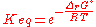which relates the equilibrium constant with Gibbs free energy.

## Standard energy change of formation

The standard Gibbs free energy of formation
Standard Gibbs function of formation
The standard Gibbs free energy of formation of a compound is the change of Gibbs free energy that accompanies the formation of 1 mole of a substance in its standard state from its constituent elements in their standard states .This table includes several Standard Gibbs...

of a compound is the change of Gibbs free energy that accompanies the formation of 1 mole
Mole (unit)
The mole is a unit of measurement used in chemistry to express amounts of a chemical substance, defined as an amount of a substance that contains as many elementary entities as there are atoms in 12 grams of pure carbon-12 , the isotope of carbon with atomic weight 12. This corresponds to a value...

of that substance from its component elements, at their standard state
Standard state
In chemistry, the standard state of a material is a reference point used to calculate its properties under different conditions. In principle, the choice of standard state is arbitrary, although the International Union of Pure and Applied Chemistry recommends a conventional set of standard states...

s (the most stable form of the element at 25 degrees Celsius and 100 kilopascals). Its symbol is ΔfG˚.

All elements in their standard states (oxygen
Oxygen
Oxygen is the element with atomic number 8 and represented by the symbol O. Its name derives from the Greek roots ὀξύς and -γενής , because at the time of naming, it was mistakenly thought that all acids required oxygen in their composition...

gas, graphite
Graphite
The mineral graphite is one of the allotropes of carbon. It was named by Abraham Gottlob Werner in 1789 from the Ancient Greek γράφω , "to draw/write", for its use in pencils, where it is commonly called lead . Unlike diamond , graphite is an electrical conductor, a semimetal...

, etc.) have 0 standard Gibbs free energy change of formation, as there is no change involved.
ΔrG = ΔrG˚ + RT ln Qr; Qr is the reaction quotient
Reaction quotient
In chemistry, a reaction quotient: Qr is a function of the activities or concentrations of the chemical species involved in a chemical reaction. In the special case that the reaction is at equilibrium the reaction quotient is equal to the equilibrium constant....

.

At equilibrium, ΔrG = 0 and Qr = K so the equation becomes ΔrG˚ = −RT ln K; K is the equilibrium constant.

### Table of selected substances

Substance State Δf(kJ/mol
Mole (unit)
The mole is a unit of measurement used in chemistry to express amounts of a chemical substance, defined as an amount of a substance that contains as many elementary entities as there are atoms in 12 grams of pure carbon-12 , the isotope of carbon with atomic weight 12. This corresponds to a value...

)
Δf(kcal
Cal
Cal may refer to:* CAL Actor Language, a dataflow language used within MPEG Reconfigurable Video Coding group to describe MPEG video decoders* Client Access License, a proprietary software license distributed by software companies such as Microsoft to allow clients to connect to its server software...

/mol
Mole (unit)
The mole is a unit of measurement used in chemistry to express amounts of a chemical substance, defined as an amount of a substance that contains as many elementary entities as there are atoms in 12 grams of pure carbon-12 , the isotope of carbon with atomic weight 12. This corresponds to a value...

)
NH3 g -12.67 −3.976
H2O l -237.13 −56.69
H2O g -174.13 −54.64
CO2 g -300.39 −94.26
CO g -104.56 −32.81
CH4 g -38.69 −12.14
C2H6 g -25.05 −7.86
C3H8 g -17.89 −5.614
C8H18 g 13.19 4.14
C10H22 g 26.23 8.23

CALPHAD stands for CALculation of PHAse Diagrams. An equilibrium phase diagram is usually a diagram with axes for temperature and composition of a chemical system. It shows the regions where substances or solutions are stable and regions where two or more of them coexist...

• Electron equivalent
Electron Equivalent
Electron Equivalent is a concept commonly used in redox chemistry, reactions involving electron transfer, to define a quantity relative to one electron. Energies of formation are often given as kilojoules per electron equivalent to enable calculation of specific reaction energies on a "per...

• Free entropy
Free entropy
A thermodynamic free entropy is an entropic thermodynamic potential analogous to the free energy. Also known as a Massieu, Planck, or Massieu-Planck potentials , or free information. In statistical mechanics, free entropies frequently appear as the logarithm of a partition function...

• Grand potential
• Thermodynamic free energy
Thermodynamic free energy
The thermodynamic free energy is the amount of work that a thermodynamic system can perform. The concept is useful in the thermodynamics of chemical or thermal processes in engineering and science. The free energy is the internal energy of a system less the amount of energy that cannot be used to...